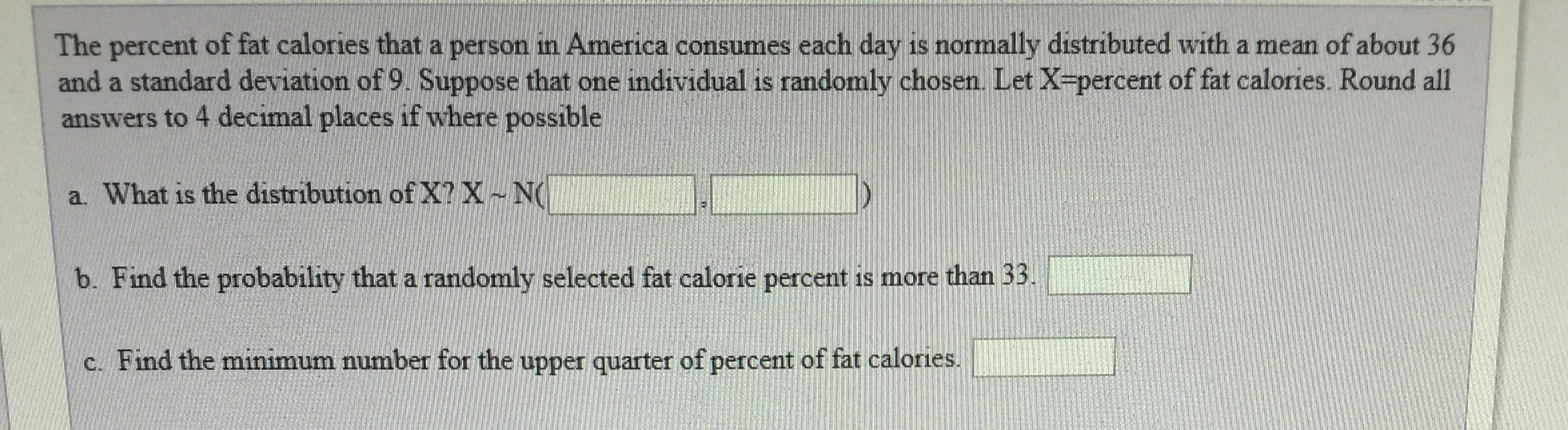# The percent of fat calories that a person in America consumes each day is normally distributed with a mean of about 36 and a standard deviation of 9 Suppose that one individual is randomly chosen Let X percent of fat calories. Round all answers to 4 decimal places if where possible a. What is the distribution of X?X ~ N( b. Find the probability that a randomly selected fat calorie percent is more than 33. c. Find the minimum number for the upper quarter of percent of fat calories.

Questionhelp_outlineImage TranscriptioncloseThe percent of fat calories that a person in America consumes each day is normally distributed with a mean of about 36 and a standard deviation of 9 Suppose that one individual is randomly chosen Let X percent of fat calories. Round all answers to 4 decimal places if where possible a. What is the distribution of X?X ~ N( b. Find the probability that a randomly selected fat calorie percent is more than 33. c. Find the minimum number for the upper quarter of percent of fat calories. fullscreen

### Want to see the step-by-step answer?